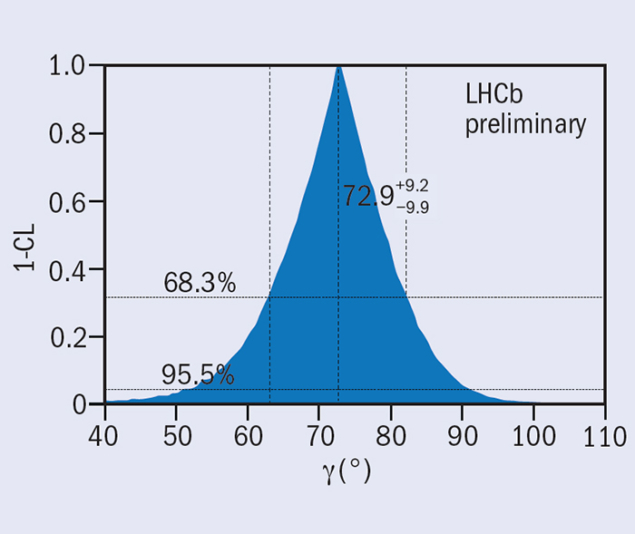# LHCb result tightens precision on angle γ

27 October 2014

For the first time in a single experiment, LHCb has achieved a precision of better than 10° in measuring the angle γ that is linked to CP violation in the Standard Model.The confidence level (CL) curve for γ from the combinations involving decays of the kind B(s) → D(s) K(*).

In the celebrated Cabibbo–Kobayashi–Maskawa (CKM) picture of three generations of quarks, the parameters that describe CP violation are constrained by one of the six triangles linked to the unitarity of the 3 × 3 quark-mixing matrix. The angles of this triangle are denoted α, β and γ, and of these it is γ that is the least precisely known. The precise measurement of γ is one of the most important goals of the LHCb experiment because it provides a powerful method to probe for the effects of new physics.

At the 8th International Workshop on the CKM Unitarity Triangle, CKM2014, which was held in Vienna recently, the LHCb collaboration presented a combination of measurements of the angle γ that yields the most precise determination so far from a single experiment. Using the full data set of 3 fb–1 integrated luminosity from the LHC running in 2011 and 2012, the collaboration has combined results on all its current measurements of “tree-level” decays. In particular, in combining results on B(s)  D(s) K(*) decays – the “robust” combination, in which a B or Bs meson decays into a D or Ds meson, respectively, and a kaon – the researchers find a best-fit value of γ = (72.9+9.2–9.9)° at the 68.3% confidence-level interval (see figure). The full combination presented at CKM2014 includes a large set of observables in B → Dπ decays that are also sensitive to γ, but to a lesser extent than the B → DK-like decays (LHCb Collaboration 2014).

Signs of new physics are not expected to show up in these tree-level decays, but they set a precise base for comparison with measurements where the observation of effects of new physics is possible. Moreover, even before taking into account data from LHC Run 2 from spring 2015, LHCb will be able to improve this result further using the data that has already been collected, because there are important analyses that are still to be completed.